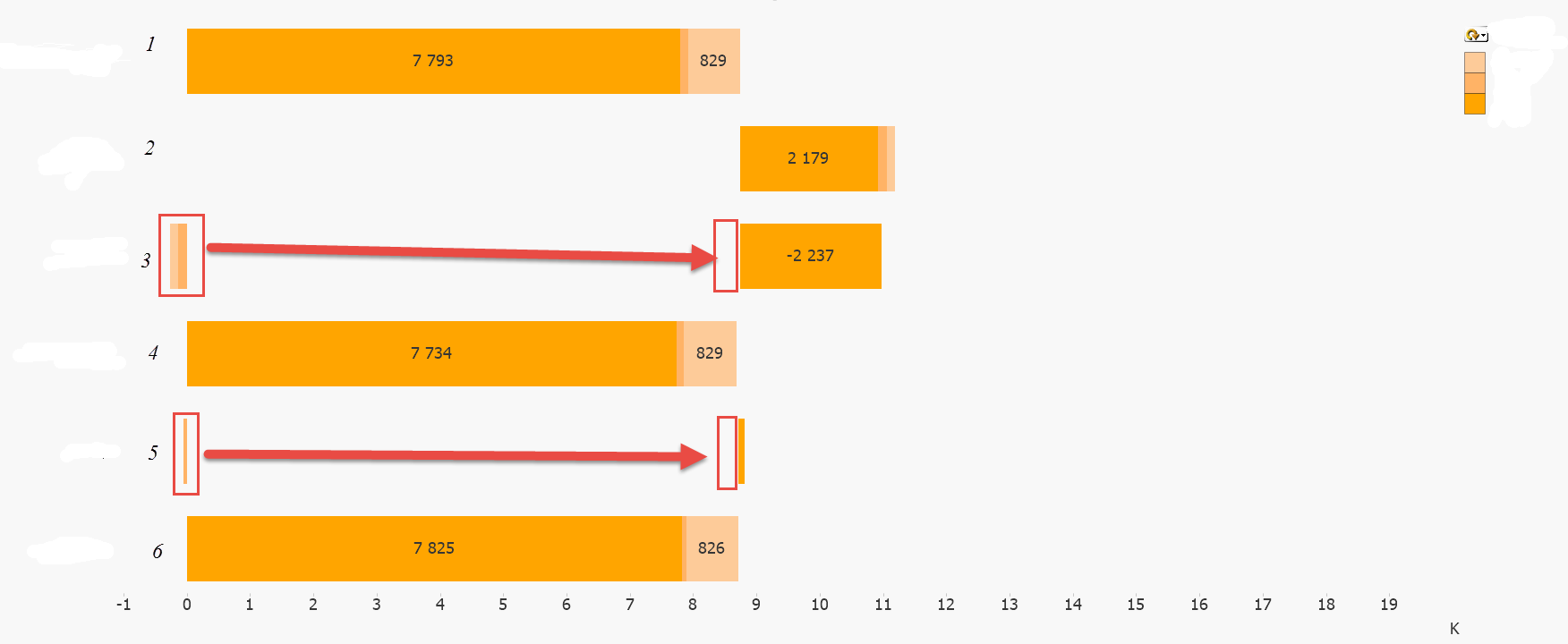QlikView App Development

Discussion Board for collaboration related to QlikView App Development.

AnnouncementsNot applicable

Issue with stacked bar offset - negative values

Hi!

I have an issue with bar offset when switching to a group to split the bar-values. My issue appears when I have negative values in the data. The first offset is set correctly - but the rest is not stacked accordingly to the first value (my arrows tells you where I want it to be):If the bars is not stacked there is no problem with the offsets and if the data has only positive values the bars are stacked fine. It's only when it contains negative numbers.

Is this possible?Bars-expression is:

if(gui_element2='1', ,

if(gui_element2='2', [2 calculated],

if(gui_element2='3', ,

if(gui_element2='4',,

if(gui_element2='5', ( - )

,

if(gui_element2='6', ,

))))))

I have calculated the bar-offset like this:

if(gui_element2='1', 0,

if(gui_element2='2', if(rowno() = 1, (sum(total {<%dim={'Com1','Com2','Com3'},yearweek={\$(vMaxYW_p)},month=,year=,week=>} fact_val)), 0),

if(gui_element2='3', if(rowno() = 1, (sum(total {<%dim={'Com1','Com2','Com3'},yearweek={\$(vMaxYW_p)},month=,year=,week=>} fact_val)), 0),

if(gui_element2='4',0,

if(gui_element2='5', if(rowno() = 1, (sum(total {<%dim={'Com1','Com2','Com3'},yearweek={\$(vMaxYW)},month=,year=,week=>} fact_val)),0),

if(gui_element2='6', 0,

))))))

Help is much appreciatedThanks

Tags (3)